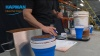Bulk density is an important calculation if you want your equipment sized correctly. Here are a few simple steps to determine your material’s bulk density.

1. Contact Hapman for a bulk density measuring cup.
2. Determine how you will collect the sample. Pulling material from the spot you will be putting new equipment is best. Bulk density can vary from depending where in the process it is gathered from.
3. Weigh the full, level cup or dump all of the contents into a sealed bag.
4. Calculate the bulk density yourself with the equations below or send the sample to us and we will calculate it for you.

W = Mass of sample and the Hapman cup together in grams.
(Remove the lid, straw, and Hapman ring)

Bulk Density = 0.0018365 * (W-138.6 g/cm3

Convert to lb/ft3 = Bulk Density (g/cm3) * 62.428 lbs/ft3

Notes: Mass of Hapman cup without lid, straw. and plastic ring = 138.7 g
Calculated Volume of Hapman Cup = 544.536 cm3 (round to 545)
Observed measured volume of Hapman Cup = approximately 550 mL